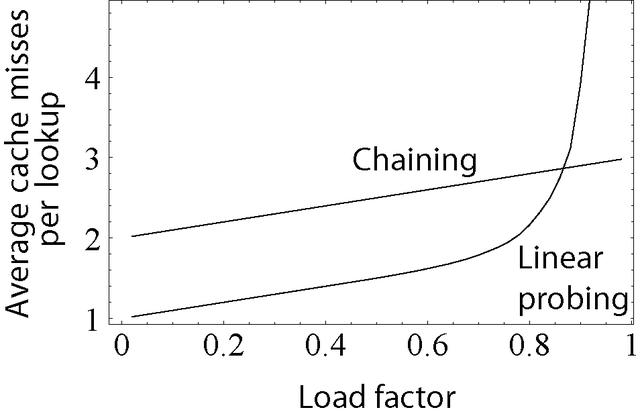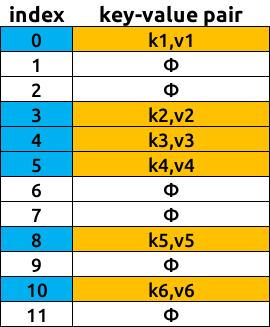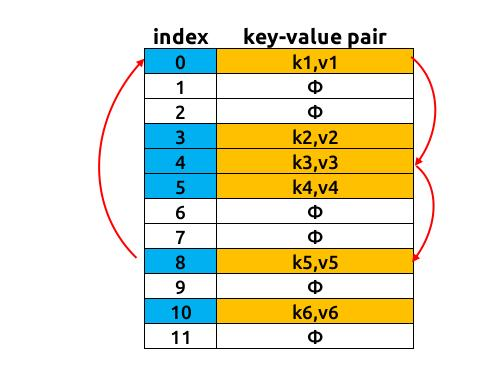# 负载系数# 探测函数

• 线性探测(Linear Probing)：p(x)= kx + b其中a，b是常数
• 二次探测(Quaratic Probing):p(x)= ax ^ 2 + bx + c，其中a，b，c是常数
• 双重散列(Double Hashing):p(k，x)= x * h(k)，其中h(k)是辅助s散列函数
• 伪随机数发生器(Pseudo Random Number Generator): p(k，x)= x*RNG(h(k),x)其中RNG是以H(k)作为种子的随机数生成器函数

# 开放寻址的通用算法

x=1keyHash=h(k)index=keyHashwhile table[index]!=NULL:      index=(keyHash+p(k,x)) mod N      x=x+1insert(k,v,index) 

# 死循环地狱(Chaos with Cycle)• 当x=2时，即index=h(k)+p(2)=(8+8) mod 12=4，探测函数会跳跃到索引4的位置，同样这里也是被占用的，如此类推
• 当x=3时,即index=h(k)+p(3)=(8+12) mod 12=8,p(x)跳跃到索引8的位置,该位置被占用
• 当x=4时,即index=h(k)+p(4)=(8+16) mod 12=0,p(x)跳跃到索引0的位置,该位置被占用
• 当x=5时,即index=h(k)+p(5)=(8+20) mod 12=4,p(x)跳跃到索引4的位置,该位置被占用
.....

# 小结

11-1208-254821
01-182万+
11-195066
08-103504
11-202985
09-083983
03-20693
11-2817
07-24641
09-043755
11-144
08-24110
09-2420
04-051万+
12-31
©️2020 CSDN 皮肤主题: 1024 设计师:白松林